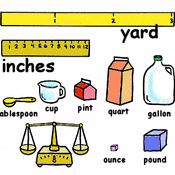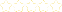• > Rachel Casto
• > Customary/Standard Measurement Conversions
+# Customary/Standard Measurement Conversions

Owner: Rachel Casto
##### Description:
Operations and Algebraic Thinking ⎯ 4.OA  A. Use the four operations with whole numbers to solve problems. 2. Multiply or divide to solve word problems involving multiplicative comparison, e.g., by using drawings and equations with a symbol for the unknown number to represent the problem, distinguishing multiplicative comparison from additive comparison.   NOTE: See Glossary, Table 2. Number and Operations in Base Ten 4.NBT  A. Generalize place value understanding for multi-digit whole numbers. 3. Use place value understanding to round multi-digit whole numbers to any place.  NOTE: Grade 4 expectations in this domain are limited to whole numbers less than or equal to 1,000,000 Measurement and Data ⎯ 4.MD  A. Solve problems involving measurement and conversion of measurements from a larger unit to a smaller unit.  2. Use the four operations to solve word problems involving distances, intervals of time, liquid volumes,masses of objects, and money, including problems involving simple fractions or decimals, and problems that require expressing measurements given in a larger unit in terms of a smaller unit. Represent measurement quantities using diagrams such as number line diagrams that feature a measurement scale.
3 Tutorials in this Playlist

#### Customary-Weight

Author: Rachel Casto
Rating:(0)

#### Customary-Length

Author: Rachel Casto
Rating:(0)

#### Customary-Capacity/Liquid Volume

Author: Rachel Casto
Rating:(0)